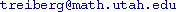Course Title: Foundations of Analysis MATH 3210 - 2 Andrejs Treibergs M, W, F, 8:35 - 9:25 AM in JTB 320 10:45 - 11:45 M, W, F, in JWB 224 (tent.)Brent Gorbutt gorbutt@math.utah.edu Calculus MATH 1210 and 1220, Advanced calculus MATH 2210 or consent of instructor. MATH 2270 (linear algebra) will be required for MATH 3220. William R. Wade, An Introduction to Analysis 3nd. Ed., Prentice Hall, 2004.

# Syllabus for Math 3210§2.

 First Homework Assignment M3210 Eleventh Homework Assignment M3210 Second Homework Assignment M3210 Twelfth Homework Assignment M3210 Third Homework Assignment M3210 Thirteenth Homework Assignment M3210 Fourth Homework Assignment M3210 Fourteenth Homework Assignment M3210 Fifth Homework Assignment M3210 Fifteenth Homework Assignment M3210 Sixth Homework Assignment M3210 Sixteenth Homework Assignment M3210 Seventh Homework Assignment M3210 Eighth Homework Assignment M3210 Ninth Homework Assignment M3210 Tenth Homework Assignment M3210

Last updated: 12 / 3 / 4